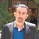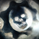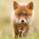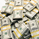1762 views
1762
EXPERIMENTAL: Experiment using Linear Regression based on %atr for decay(decay option is a mutiplier for the atr).
```study("[RS]Decay Channel Candles V0", overlay=true)
linreg_length = input(type=integer, defval=4)
atr_length = input(type=integer, defval=100)
decay = atr(atr_length)*input(type=float, defval=0.125)
prev_top = nz(top, high)-decay
prev_bot = nz(bot, low)+decay
abs_top = valuewhen(high >= prev_top, high, 0)
abs_bot = valuewhen(low <= prev_bot, low, 0)
top = high >= prev_top ? high : prev_top
bot = low <= prev_bot ? low : prev_bot
top_close = max(high, min(top, linreg(avg(top, high), linreg_length, 0)))
bot_close = min(low, max(bot, linreg(avg(bot, low), linreg_length, 0)))
//#9ce0b2//#e0b29c
top_palete = rising(abs(top-top_close),1)?orange:#e0b29c
bot_palete = rising(abs(bot-bot_close),1)?olive:#9ce0b2
plotbar(top,top,top,top_close, color=top_palete)
plotbar(bot,bot,bot,bot_close, color=bot_palete)
plot(abs_top, color=abs_top==abs_top?maroon:na)
plot(abs_bot, color=abs_bot==abs_bot?green:na)

```Thanks for sharing,
I have quick question: how to draw lines ?

Draw_line(price1, candle1, price2, candle2)

how to achieve this function ?
AntwortenGood indicator, for an Options trader. How and when to manage positions. Etc. Good job. Keep it flowing.......
Antwortenit uses linear regression to map breakouts, on tight ranges you would need to accommodate the volume of decay manually to adjust to the tighter volatility(usually on accumulation zones but not limited as high volume volatility can result on the same effect), for clarification this is a lagging indicator and its purpose should be to give a better understanding of the underlying movement.
AntwortenRicardoSantos
thats great thank you Ricardo now let me dig deeper appreciate chart and explanation
AntwortenHey Richardo can you give an explanation and your thought process for fuller understanding ..thank you
AntwortenHi Ricardo, could you elaborate on how to read the indicator? Thanks
Antworten